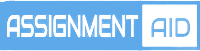# Data Mining And Visualization Assignment#### Data Mining And Visualization Assignment

1. Dimension Reduction (5%) This item requires the dataset Utilities.xls which can be found on the subject Interact site. This dataset gives corporate data on 22 US public utilities. We are interested in forming groups of similar utilities. The objects to be clustered are the utilities. There are 8 measurements on each utility described below. An example where clustering would be useful is a study to predict the cost impact of deregulation. To do the requisite analysis economists would need to build a detailed cost model of the various utilities. It would save a considerable amount of time and effort if we could cluster similar types of utilities and to build detailed cost models for just one ”typical” utility in each cluster and then scaling up from these models to estimate results for all utilities. The objects to be clustered are the utilities and there are 8 measurements on each utility.

X1: Fixed-charge covering ratio (income/debt) X2: Rate of return on capital X3: Cost per KW capacity in place X4: Annual Load Factor X5: Peak KWH demand growth from 1974 to 1975 X6: Sales (KWH use per year) X7: Percent Nuclear X8: Total fuel costs (cents per KWH)

a. Conduct Principal Component Analysis (PCA) on the data. Evaluate and comment on the Results. Should the data be normalized? Discuss what characterizes the components you consider key and justify your answer. b. Briefly explain advantages and any disadvantages of using the PCA compared to other methods for this task.

2. Naïve Bayes Classifier (10%)

This item requires the dataset UniversalBank.xls which can be found on the subject Interact site. The following is a business analytical problem faced by financial institutions and banks. The objective is to determine the measurements for personal loan acceptance. The dataset UniversalBank.xls contains data on 5000 customers of Universal Bank. The data include customer demographic information (age, income, etc.), the customer’s relationship with the bank (mortgage, securities account etc.), and the customer response to the last personal loan campaign (Personal Loan). Among these 5000 customers, only 480 (= 9.6%) accepted the personal loan that was offered to them in the earlier campaign. In this exercise we focus on two predictors: Online (whether or not the customer is an active user of online banking services) and Credit Card (abbreviated CC below) (does the customer hold a credit card issued by the bank), and the outcome Personal Loan (abbreviated Loan below). Partition the data into training (60%) and validation (40%) sets.

a. Create a pivot table for the training data with Online as a column variable, CC as a row variable, and Loan as a secondary row variable. The values inside the cells should convey the count (how many records are in that cell). b. Consider the task of classifying a customer who owns a bank credit card and is actively using online banking services. Analyse the pivot table and calculate the probability that this customer will accept the loan offer. Note: This is the probability of loan acceptance (Loan=1) conditional on having a bank credit card (CC=1) and being an active user of online banking services (Online=1). c. Design two separate pivot tables for the training data. One will have Loan (rows) as a function of Online (columns) and the other will have Loan (rows) as a function of CC. Compute the following quantities [P(A | B) means “the probability of A given B”]: i. P(CC=1 | Loan=1) (the proportion of credit card holders among the loan acceptors) ii. P(Online=1 | Loan=1) iii. P(Loan=1) (the proportion of loan acceptors) iv. P(CC=1 | Loan=0) v. P(Online=1 | Loan=0) vi. P(Loan=0) d. Using the quantities computed in (c), compute the Naive Bayes probability P(Loan=1 | CC=1, Online=1). e. Based on the calculations above, suggest the best possible strategy for the customer to get the loan.

Rationale

This task assesses your progress towards meeting Learning Outcomes 1, 2 and 3: 1. Be able to identify and analyse business requirements for the identification of patterns and trends in data sets 2. Be able to appraise the different approaches and categories of data mining problems. 3. Be able to compare and evaluate output patterns

## Example Samples

live:topwrit

###### Email:

support@assignmentsaid.com

###### Open Hours:

Mon-Sat: 9am - 6pm